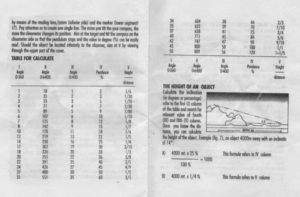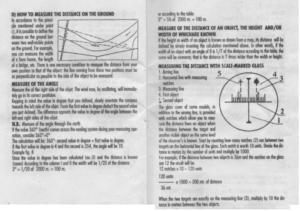Categories

# Compass and Clinometer Measurements.

As promised in the previous post, some scans on the angle measuring instructions that came with my “Italian Army” compass.The first paragraph refers to the clinometer. If you work in certain fields the contents of column V will be familiar to you. This column can be read as either fractions or gradients. That is, for example, 25% = ¼ = 1 in 4. A slope of 14° therefore drops or rises one metre for every four metres of horizontal distance. A 45° slope is 1/1 so changes by a metre for every horizontal metre.
In the illustrated example below a hilltop is measures as being at 14° to the viewer so its height must be a quarter of the distance between the two. The converse is also true. If you know the height of an object and can measure the angle you can calculate the distance. A building storey is 3 metres so a three story building will be 9 metres. If the observed angle to the top of the third storey is 6° then the distance must be 90 (9 x 10) metres.
The same system can be used with horizontal angles, taken from bearings of each side of an object. Also illustrated is a way to use the graduations on the window in the cover to calculate the distance from an object of known width.